# Demystifying delayed aesthetic evaluation: Part 2

Exposing the `Stat` ggproto in functional programming terms

June Choe (University of Pennsylvania Linguistics)https://live-sas-www-ling.pantheon.sas.upenn.edu/
2022-07-06

This is a developing series of blog posts, scheduled for three parts:

## Introduction

Let’s pick up where we left off in Part 1. If you’d like to follow along without going back to re-read it, the relevant code that we’ll carry over here is provided below:

Setup from Part 1
``````# Top-level setup
library(ggplot2)
library(dplyr)
library(palmerpenguins)

set.seed(2022)
theme_set(theme_classic(13))

# Custom function to inspect `after_stat()`
inspect_after_stat <- function(p, i = 1L) {
._env <- environment()
.out <- NULL
suppressMessages({
trace(
what = "ggplot_build.ggplot",
tracer = substitute(assign(".out", data[[i]], envir = ._env), ._env),
at = 19L,
print = FALSE,
where = asNamespace("ggplot2")
)
})
ggplot_build(p)
suppressMessages({
untrace("ggplot_build.ggplot", where = asNamespace("ggplot2"))
})
.out
}
``````

To recap, the big theme of Part 1 was about how each layer of a ggplot transforms the raw data under the hood to make it “drawing ready” (i.e., the dataframe returned by `layer_data()`). We saw that a lot happens over the course of this data transformation pipeline, one of which is the statistical transformation step. For example, the `geom_bar()` layer uses `stat = "count"` (shorthand for `stat = StatCount`) by default, which computes new variables like `count` and `prop` internally.

We also saw how `after_stat()` allows users to declare a delayed aesthetic mapping, which waits to be applied until after this statistical transformation step. For example, the `StatCount` stat used by `geom_bar()` specifies the default implicit mapping to `after_stat(count)`.

Objects like `StatCount` are called ggproto objects, and they’re the focus of this Part 2 of the series. We’ll be digging into the implementational details of the internal statistical transformation step. Along the way, we’ll encounter some funny looking functions in the form of `<obj>\$<method>()`. These are called ggproto methods - they look pretty scary, but needlessly so for our purposes: aside from their odd syntax, most of them are essentially just data wrangling functions that we’re already familiar with.

Let’s dive right in!

## Where it all happens: `Stat\$compute_layer()`

Let’s again use the `penguins` dataset and visualize penguin species counts with `geom_bar()`. This time we’ll also give the bars `width = 0.7` and facet by island:

``````library(palmerpenguins)
p_bar2 <- ggplot(penguins, aes(x = species)) +
geom_bar(width = 0.7) +
facet_wrap(~ island)
p_bar2
``````As expected, `geom_bar()` maps the internally computed `count` variable to the `y` aesthetic by default. We suspect that something like `{dplyr}`’s `group_by()` and `summarize()` (or just `count()`) is happening in the statistical transformation stage:

``````penguins %>%
group_by(island, species) %>%
summarize(count = n(), .groups = "drop")
``````
``````  # A tibble: 5 × 3
island    species   count
<fct>     <fct>     <int>
2 Biscoe    Gentoo      124
4 Dream     Chinstrap    68
``````

In Part 1, I introduced this mystery function called `inspect_after_stat()` to show you that this is indeed the case:

``````inspect_after_stat(p_bar2)
``````
``````    count prop x width flipped_aes PANEL group
1    44    1 1   0.7       FALSE     1     1
2   124    1 3   0.7       FALSE     1     3
3    56    1 1   0.7       FALSE     2     1
4    68    1 2   0.7       FALSE     2     2
5    52    1 1   0.7       FALSE     3     1
``````

Now it’s time to unveil the mystery behind this function - `inspect_after_stat()` was grabbing the return value of the ggproto method `Stat\$compute_layer()` when it was called for the first layer of our plot.1

Using the function `ggtrace_inspect_return()` from my package `{ggtrace}`, we can achieve this more explicitly. We pass the function our plot and the ggproto method we want to inspect, and it gives us what the method returned:

``````# install.packages("remotes")
# remotes::install_github("yjunechoe/ggtrace")
library(ggtrace)
compute_layer_output <- ggtrace_inspect_return(p_bar2, Stat\$compute_layer)
compute_layer_output
``````
``````    count prop x width flipped_aes PANEL group
1    44    1 1   0.7       FALSE     1     1
2   124    1 3   0.7       FALSE     1     3
3    56    1 1   0.7       FALSE     2     1
4    68    1 2   0.7       FALSE     2     2
5    52    1 1   0.7       FALSE     3     1
``````

If ggproto methods are essentially functions, they should have inputs and outputs. We just saw the output of `Stat\$compute_layer()`, but what’s its input?

We can simply swap out `ggtrace_inspect_return()` with `ggtrace_inspect_args()` to look at the arguments that it was called with:

``````compute_layer_input <- ggtrace_inspect_args(p_bar2, Stat\$compute_layer)
names( compute_layer_input )
``````
``````   "self"   "data"   "params" "layout"
``````

We can ignore the `self` and `layout` arguments for the moment. The crucial ones are the `data` and `params` arguments, which look like this:

``````compute_layer_input\$data
``````
``````compute_layer_input\$params
``````
``````  \$width
 0.7

\$na.rm
 FALSE

\$orientation
 NA

\$flipped_aes
 FALSE
``````

Remember how I said ggproto methods are essentially data wrangling functions? We can think of `Stat\$compute_layer()` as a function that takes a dataframe and a list of parameters, and does a simple data wrangling after grouping by the `PANEL` and `group` columns:

``````compute_layer_fn <- function(data, params) {
data %>%
group_by(PANEL, group) %>%
summarize(
count = n(),
x = unique(x),
width = params\$width,
flipped_aes = params\$flipped_aes,
.groups = 'drop'
) %>%
relocate(PANEL, group, .after = last_col())
}
``````

This gets us the a dataframe that’s very similar to the output of `Stat\$compute_layer()`:

``````compute_layer_fn(compute_layer_input\$data, compute_layer_input\$params)
``````
``````  # A tibble: 5 × 6
count     x width flipped_aes PANEL group
<int> <int> <dbl> <lgl>       <fct> <int>
1    44     1   0.7 FALSE       1         1
2   124     3   0.7 FALSE       1         3
3    56     1   0.7 FALSE       2         1
4    68     2   0.7 FALSE       2         2
5    52     1   0.7 FALSE       3         1
``````

So we see that the `Stat\$compute_layer()` method was the step in the internals responsible for calculating the statistical summaries necessary to draw our bar layer.

But there’s a catch - the way that the method is written doesn’t directly reflect this. Nothing about the code for `Stat\$compute_layer()` says anything about counting:

``````Stat\$compute_layer
``````
``````  function (self, data, params, layout)
{
check_required_aesthetics(self\$required_aes, c(names(data),
names(params)), snake_class(self))
required_aes <- intersect(names(data), unlist(strsplit(self\$required_aes,
"|", fixed = TRUE)))
data <- remove_missing(data, params\$na.rm, c(required_aes,
self\$non_missing_aes), snake_class(self), finite = TRUE)
params <- params[intersect(names(params), self\$parameters())]
args <- c(list(data = quote(data), scales = quote(scales)),
params)
dapply(data, "PANEL", function(data) {
scales <- layout\$get_scales(data\$PANEL)
tryCatch(do.call(self\$compute_panel, args), error = function(e) {
warn(glue("Computation failed in `{snake_class(self)}()`:\n{e\$message}"))
new_data_frame()
})
})
}
<bytecode: 0x000002e49ae09aa0>
<environment: namespace:ggplot2>
``````

Where’s the relevant calculation actually happening? For that, we need to go deeper.

## Where it specifically happens: `StatCount\$compute_group()`

The actual calculation of counts for the bar layer happens inside another ggproto method called `StatCount\$compute_group()`:

``````StatCount\$compute_group
``````
``````  function (self, data, scales, width = NULL, flipped_aes = FALSE)
{
data <- flip_data(data, flipped_aes)
x <- data\$x
weight <- data\$weight %||% rep(1, length(x))
count <- as.numeric(tapply(weight, x, sum, na.rm = TRUE))
count[is.na(count)] <- 0
bars <- new_data_frame(list(count = count, prop = count/sum(abs(count)),
x = sort(unique(x)), width = width, flipped_aes = flipped_aes),
n = length(count))
flip_data(bars, flipped_aes)
}
<bytecode: 0x000002e49b824e38>
<environment: namespace:ggplot2>
``````

We don’t need to dwell on trying to understand the code - let’s dive straight in with the same `ggtrace::ggtrace_inspect_*()` functions.

We see that the return value is actually just one row of the return value of `Stat\$compute_layer()` that we saw earlier, minus the `PANEL` and `group` columns:2

``````ggtrace_inspect_return(p_bar2, StatCount\$compute_group)
``````
``````    count prop x width flipped_aes
1    44    1 1   0.7       FALSE
``````

As the name suggests, `\$compute_group()` is called for each group (in this case, bar) in the `geom_bar()` layer. The method is called after the layer’s data is split by facet and group, much like how we used `group_by(PANEL, group)` to simulate `compute_layer_fn()` above.

Using `ggtrace_inspect_n()` which returns how many times a ggproto method was called in a plot, we confirm that `StatCount\$compute_group()` was indeed called five times, once for each bar in the plot:

``````ggtrace_inspect_n(p_bar2, StatCount\$compute_group)
``````
``````   5
``````

And we can (mostly) recover the output of `Stat\$compute_layer()` by combining the return values from `StatCount\$compute_group()`, all five times it’s called for the layer. The `cond` argument here lets us target the nth time the method is called:

``````bind_rows(
ggtrace_inspect_return(p_bar2, StatCount\$compute_group, cond = 1),
ggtrace_inspect_return(p_bar2, StatCount\$compute_group, cond = 2),
ggtrace_inspect_return(p_bar2, StatCount\$compute_group, cond = 3),
ggtrace_inspect_return(p_bar2, StatCount\$compute_group, cond = 4),
ggtrace_inspect_return(p_bar2, StatCount\$compute_group, cond = 5)
)
``````
``````    count prop x width flipped_aes
1    44    1 1   0.7       FALSE
2   124    1 3   0.7       FALSE
3    56    1 1   0.7       FALSE
4    68    1 2   0.7       FALSE
5    52    1 1   0.7       FALSE
``````

So we get a sense that `Stat\$compute_layer()` simply splits the layer’s data by panel and group, while `StatCount\$compute_group()` does the heavy lifting.

But what’s the relationship between these two ggproto methods? How does `Stat\$compute_layer()` know to use `StatCount\$compute_group()`?

Long story short, the statistical transformation step for `geom_bar()` is actually ALL about `StatCount`. So `Stat\$compute_layer()` is essentially `StatCount\$compute_layer()`, but also kind of not.

To understand this distinction fully, we need a slight detour into the world of ggproto.

## `ggproto`, minus the “gg” and the “proto”

There are existing resources for learning how ggproto works, such as Chapter 20 of the ggplot2 book, so I won’t repeat all the details here. In fact, if you’re already familiar with object-oriented programming, the book chapter has all you need. But you’re like me and found it overwhelming at first, then this is for you!

On notations and conventions

### My conventions

In this blog post, I’ll refer to ggproto methods like `\$method()` to distinguish it from normal functions. I’ll refer to properties (non-function elements of ggproto objects) like `\$property` to distinguish it from variables.

Also, whenever I’m printing ggproto methods, I’m actually using `get("method", object)` behind the scenes. I do this to bring attention to the method body - printing ggproto methods as-is comes with extra baggage that distracts from the topic of this blog post. Just be aware of this when you go exploring ggproto methods yourself:

``````# This just prints the method body
get("setup_data", Stat)
``````
``````  function (data, params)
{
data
}
<environment: namespace:ggplot2>
``````
``````# Prints extra stuff we don't care about
# - see: `ggplot2:::format.ggproto_method`
Stat\$setup_data
``````
``````  <ggproto method>
<Wrapper function>
function (...)
f(...)

<Inner function (f)>
function (data, params)
{
data
}
``````

### {ggplot2} conventions

You might have noticed how ggproto objects are written in upper camel case like `StatCount`. This is by convention, but they’re very important to know and follow. The upper camel case convention for ggproto objects is closely related to two other conventions:

• The first is in the naming of the layer functions we use to write ggplot code and chain with the `+` operator, like `geom_bar()` and `stat_count()`. These names are derived from ggproto objects like `GeomBar` and `StatCount` through the internal function `ggplot2:::snake_class()`. Thus, the camel case convention is used to distinguish ggproto objects like `StatCount` from constructor functions like `stat_count()`, while maintaining a predictable connection between the two.

• The second is in the use of character shorthands to refer to ggproto objects inside layer functions, like `geom_bar(stat = "count")`. These shorthands work by turning the string into upper camel case using the internal function `ggplot2:::camelize(x, first = TRUE)` and then prefixing that with `"Stat"` (or `"Geom"` or `"Position"`). So `"count"` gets converted into `"StatCount"`, which then gets looked up in the caller environment. If a ggproto object breaks this convention, the character shorthand would not work.

Relatedly, note how variable names like `StatCount` match the class name `class(StatCount)`:

``````class(StatCount)
``````
``````   "StatCount"
``````

This is also by convention and it’s useful for figuring out what specific `Stat`/`Geom`/`Position` a layer uses:

``````class( geom_bar()\$stat )      # uses `StatCount` ggproto
``````
``````   "StatCount"
``````
``````class( geom_bar()\$geom )      # uses `GeomBar` ggproto
``````
``````   "GeomBar"
``````
``````class( geom_bar()\$position )  # uses `PositionStack` ggproto
``````
``````   "PositionStack"
``````

When we distill it down to the very basics, ggprotos are essentially lists, and ggproto methods are essentially functions. For our purposes, the only truly new concept you need to know about ggproto methods is that they’re functions that live inside lists.

So `StatCount\$compute_group()` calls the `\$compute_group()` function defined inside a list called `StatCount`, kind of like this:

``````# Not run
StatCount <- list(
compute_group = function(...) { ... },
...
)
``````

And `Stat\$compute_layer()` calls the `\$compute_layer()` function defined inside a list called `Stat`:

``````# Not run
Stat <- list(
compute_layer = function(...) { ... },
...
)
``````

The reason why the layer-level statistical transformation for `geom_bar()` was handled by `Stat\$compute_layer()` and not `StatCount\$compute_layer()` is just by technicality. In fact, `StatCount\$compute_layer()` does “exist”:

``````StatCount\$compute_layer
``````
``````  function (self, data, params, layout)
{
check_required_aesthetics(self\$required_aes, c(names(data),
names(params)), snake_class(self))
required_aes <- intersect(names(data), unlist(strsplit(self\$required_aes,
"|", fixed = TRUE)))
data <- remove_missing(data, params\$na.rm, c(required_aes,
self\$non_missing_aes), snake_class(self), finite = TRUE)
params <- params[intersect(names(params), self\$parameters())]
args <- c(list(data = quote(data), scales = quote(scales)),
params)
dapply(data, "PANEL", function(data) {
scales <- layout\$get_scales(data\$PANEL)
tryCatch(do.call(self\$compute_panel, args), error = function(e) {
warn(glue("Computation failed in `{snake_class(self)}()`:\n{e\$message}"))
new_data_frame()
})
})
}
<bytecode: 0x000002e49ae09aa0>
<environment: namespace:ggplot2>
``````

But only in a very specific sense - it just recycles the `\$compute_layer()` function defined in `Stat`, similar to in this implementation:

``````# Not run
StatCount <- list(
compute_group = function() { ... },
compute_layer = Stat\$compute_layer,
...
)
``````

In object-orientated programming terms, this is called inheritance - the `StatCount` ggproto is a child of the parent `Stat` ggproto that inherits some of the parent’s methods (like `\$compute_layer()`) while overriding and defining some of its own (like `\$compute_group()`).

A note on class inheritance

Class inheritance is reflected in the output of `class()`, where order of elements matter:

``````class(Stat)
``````
``````   "Stat"    "ggproto" "gg"
``````
``````class(StatCount) # see also: `inherits(StatCount, "Stat")`
``````
``````   "StatCount" "Stat"      "ggproto"   "gg"
``````

By design, all `Stat*` ggprotos inherit from the top-level parent `Stat` ggproto. For example, the `geom_boxplot()` layer uses `StatBoxplot` for its stat, which also inherits from `Stat`:

``````class( geom_boxplot()\$stat ) # or `class(StatBoxplot)`
``````
``````   "StatBoxplot" "Stat"        "ggproto"     "gg"
``````

Sometimes, an inheritance chain can be more complex - for example, `StatDensity2dFilled` inherits from `StatDensity2d`, which in turn inherits from `Stat`:

``````class( StatDensity2dFilled )
``````
``````   "StatDensity2dFilled" "StatDensity2d"       "Stat"
 "ggproto"             "gg"
``````

But what’s the point of all this? Why do we bother with these clunky ggproto objects instead of having a single function that does all bar-related things, all boxplot-related things, etc.?

## Templates and extensions

The rationale behind this inheritance-based design is that the top-level `Stat` ggproto serves as a template that’s meant to be filled and customized.

Another term for this customization is extension - for example, we say that `StatCount` is an extension of `Stat`. This is what’s technically meant by “ggplot2 extension packages” - these packages provide new `Stat*` or `Geom*` ggproto objects (like `ggforce::StatSina` and `ggtext::GeomRichtext`) that are extensions of the top-level `Stat` and `Geom` ggprotos.

Since `Stat` is essentially a list and extensions are essentially a way of customizing certain elements of a template, each element of `Stat` can be thought of as a possible extension point:

``````names(Stat)
``````
``````    "compute_layer"   "parameters"      "aesthetics"      "setup_data"
 "retransform"     "optional_aes"    "non_missing_aes" "default_aes"
 "finish_layer"    "compute_panel"   "extra_params"    "compute_group"
 "required_aes"    "setup_params"
``````

Some extension points are “methods” (functions) and others are “properties” (non-functions):

``````sapply(Stat, class)
``````
``````    compute_layer      parameters      aesthetics      setup_data     retransform
"function"      "function"      "function"      "function"       "logical"
optional_aes non_missing_aes     default_aes    finish_layer   compute_panel
"character"     "character"        "uneval"      "function"      "function"
extra_params   compute_group    required_aes    setup_params
"character"      "function"     "character"      "function"
``````

If you want to know what each of these methods and properties are for, you can read up on the package vignette on ggproto. But we don’t need to know every detail - only a handful are productive extension points.

Below are a few examples of specific `Stat*` extensions (e.g., `StatCount`) and information about what methods/properties they modify from the top-level `Stat` ggproto:

Try to get a feel for what kind of methods and properties are the common targets of extensions. Pay specific attention to the distribution of methods highlighted in green.

## `StatCount`

``````get_method_inheritance(StatCount)
``````
``````  \$Stat
 "aesthetics"      "compute_layer"   "compute_panel"   "finish_layer"
 "non_missing_aes" "optional_aes"    "parameters"      "retransform"
 "setup_data"

\$StatCount
 "compute_group" "default_aes"   "extra_params"  "required_aes"
 "setup_params"
``````

## `StatBoxplot`

``````get_method_inheritance(StatBoxplot)
``````
``````  \$Stat
 "aesthetics"    "compute_layer" "compute_panel" "default_aes"
 "finish_layer"  "optional_aes"  "parameters"    "retransform"

\$StatBoxplot
 "compute_group"   "extra_params"    "non_missing_aes" "required_aes"
 "setup_data"      "setup_params"
``````

## `StatDensity`

``````get_method_inheritance(StatBin)
``````
``````  \$Stat
 "aesthetics"      "compute_layer"   "compute_panel"   "finish_layer"
 "non_missing_aes" "optional_aes"    "parameters"      "retransform"
 "setup_data"

\$StatBin
 "compute_group" "default_aes"   "extra_params"  "required_aes"
 "setup_params"
``````

## `StatSmooth`

``````get_method_inheritance(StatSmooth)
``````
``````  \$Stat
 "aesthetics"      "compute_layer"   "compute_panel"   "default_aes"
 "finish_layer"    "non_missing_aes" "optional_aes"    "parameters"
 "retransform"     "setup_data"

\$StatSmooth
 "compute_group" "extra_params"  "required_aes"  "setup_params"
``````

## `StatBin`

``````get_method_inheritance(StatBin)
``````
``````  \$Stat
 "aesthetics"      "compute_layer"   "compute_panel"   "finish_layer"
 "non_missing_aes" "optional_aes"    "parameters"      "retransform"
 "setup_data"

\$StatBin
 "compute_group" "default_aes"   "extra_params"  "required_aes"
 "setup_params"
``````

## `StatBin`

``````get_method_inheritance(StatContour)
``````
``````  \$Stat
 "aesthetics"      "compute_layer"   "compute_panel"   "extra_params"
 "finish_layer"    "non_missing_aes" "optional_aes"    "parameters"
 "retransform"     "setup_data"

\$StatContour
 "compute_group" "default_aes"   "required_aes"  "setup_params"
``````

Did you notice how all these `Stat*` extensions define their own `\$compute_group()` method while inheriting the `\$compute_layer()` and `\$compute_panel()` methods from the parent `stat`? This is the intended design - check out how `Stat\$compute_group()` is defined:

``````Stat\$compute_group
``````
``````  function (self, data, scales)
{
abort("Not implemented")
}
<bytecode: 0x000002e49ae09838>
<environment: namespace:ggplot2>
``````

And this is what I mean by “the top-level `Stat` ggproto is a template”. The `Stat` provides an infrastructure that splits the data up by layer (`Stat\$compute_layer()`) and panel (`Stat\$compute_panel()`), but leaves it to the child `Stat*` ggprotos to fill in the details about what statistical summaries are computed by group, after this splitting takes place.

How are `Stat\$compute_layer()` and `Stat\$compute_panel()` implemented?

In `\$compute_layer()`, the data is split by values of the `PANEL` column using an internal function `ggplot2:::dapply()`, and `\$compute_panel()` is called on each split.

``````Stat\$compute_layer
``````
``````  function (self, data, params, layout)
{
check_required_aesthetics(self\$required_aes, c(names(data),
names(params)), snake_class(self))
required_aes <- intersect(names(data), unlist(strsplit(self\$required_aes,
"|", fixed = TRUE)))
data <- remove_missing(data, params\$na.rm, c(required_aes,
self\$non_missing_aes), snake_class(self), finite = TRUE)
params <- params[intersect(names(params), self\$parameters())]
args <- c(list(data = quote(data), scales = quote(scales)),
params)
dapply(data, "PANEL", function(data) {
scales <- layout\$get_scales(data\$PANEL)
tryCatch(do.call(self\$compute_panel, args), error = function(e) {
warn(glue("Computation failed in `{snake_class(self)}()`:\n{e\$message}"))
new_data_frame()
})
})
}
<bytecode: 0x000002e49ae09aa0>
<environment: namespace:ggplot2>
``````

In `\$compute_panel()`, the data is first split by values of the `group` column using the `split()` function, then `\$compute_group()` is called on each split inside `lapply()`.

``````Stat\$compute_panel
``````
``````  function (self, data, scales, ...)
{
if (empty(data))
return(new_data_frame())
groups <- split(data, data\$group)
stats <- lapply(groups, function(group) {
self\$compute_group(data = group, scales = scales, ...)
})
stats <- mapply(function(new, old) {
if (empty(new))
return(new_data_frame())
unique <- uniquecols(old)
missing <- !(names(unique) %in% names(new))
cbind(new, unique[rep(1, nrow(new)), missing, drop = FALSE])
}, stats, groups, SIMPLIFY = FALSE)
rbind_dfs(stats)
}
<environment: namespace:ggplot2>
``````

These two methods are implemented slightly differently, though the exact details are not relevant to the current discussion.3

Lastly, a note about `self`. The `self` variable is a reference to the ggproto object that called the method.4 In the context of `p_bar2`, the `self` is `StatCount`:

``````class( ggtrace_inspect_args(p_bar2, Stat\$compute_layer)\$self )
``````
``````   "StatCount" "Stat"      "ggproto"   "gg"
``````

This may be surprising given how `\$compute_layer()` lives inside `Stat`, not `StatCount`. This is an odd thing about object oriented programming with ggproto that you’ll have to get used to. Just remember that the choice of the ggproto object is determined by the layer (e.g., stored in `geom_bar()\$stat`) and that the `self` variable keeps track of which ggproto object called a method (context-dependent); but all of this is separate from the issue of where a method is defined in (context-independent).

In other words, `self` is `StatCount` because the method `\$compute_layer()` is “looked up” by `StatCount`. The fact that the actual `\$compute_layer()` method is defined inside `Stat\$compute_layer()` is irrelevant here (though it may be relevant for other purposes).

In the next section, we’ll look at how the `\$compute_*()` family of methods behave in the internals.

Wait - what about the other common extension points?

You might have also noticed a few more repeated extension points other than `\$compute_group()`. They usually form some subset of `\$default_aes`, `\$required_aes`, `\$setup_data()`, `\$setup_params()`, and `\$extra_params`. These are also like a family - their job is to prepare the layer’s data before it’s sent off to the `\$compute_*()` family of methods.

For example, here’s a walkthrough of how `StatCount` prepares the data:

First, the default aesthetic mappings are specified such that both `x` and `y` are mapped to `after_stat(count)`:

``````# or `geom_bar()\$stat\$default_aes`
StatCount\$default_aes
``````
``````  Aesthetic mapping:
* `x`      -> `after_stat(count)`
* `y`      -> `after_stat(count)`
* `weight` -> 1
``````

Second, the required aesthetic mappings are specified such that exactly one of `x` or `y` must be provided:

``````# or `geom_bar()\$stat\$required_aes`
StatCount\$required_aes
``````
``````   "x|y"
``````

By requiring the user to supply one of the two aesthetics, the one left over takes on the “implicit” value of `after_stat(count)`. This is largely handled in `StatCount\$setup_params()`:

``````# Lots of code here but it does 3 things:
# 1) Check if user supplied `y`, not `x` (+ track this in `flipped_aes`)
# 2) Make sure user supplied exactly one of `x` or `y`
# 3) If `flipped_aes` (= `y` is supplied), pretend that it's `x` but
#    keep that for later (reverted in `StatCount\$compute_group()`)
StatCount\$setup_params
``````
``````  function (data, params)
{
params\$flipped_aes <- has_flipped_aes(data, params, main_is_orthogonal = FALSE)
has_x <- !(is.null(data\$x) && is.null(params\$x))
has_y <- !(is.null(data\$y) && is.null(params\$y))
if (!has_x && !has_y) {
abort("stat_count() requires an x or y aesthetic.")
}
if (has_x && has_y) {
abort("stat_count() can only have an x or y aesthetic.")
}
if (is.null(params\$width)) {
x <- if (params\$flipped_aes)
"y"
else "x"
params\$width <- resolution(data[[x]]) * 0.9
}
params
}
<bytecode: 0x000002e49b8212e8>
<environment: namespace:ggplot2>
``````

Lastly, the role of `\$extra_params` is kind of weird, but in this case it simply says that `StatCount` has support for handling different orientations (which gets standardized to `flipped_aes` internally).

``````Stat\$extra_params
``````
``````   "na.rm"
``````
``````StatCount\$extra_params
``````
``````   "na.rm"       "orientation"
``````

In case you didn’t know already, `orientation` is a relatively new (v3.3.0) argument supported by some layers. It’s allows individual layers to be “flipped” without applying `coord_flip()` to the whole plot:

``````ggplot(mapping = aes(x = 2, y = 3)) +
geom_col(fill = "pink") +
geom_col(fill = "steelblue", orientation = "y") +
xlim(0, 3) + ylim(0, 4)
``````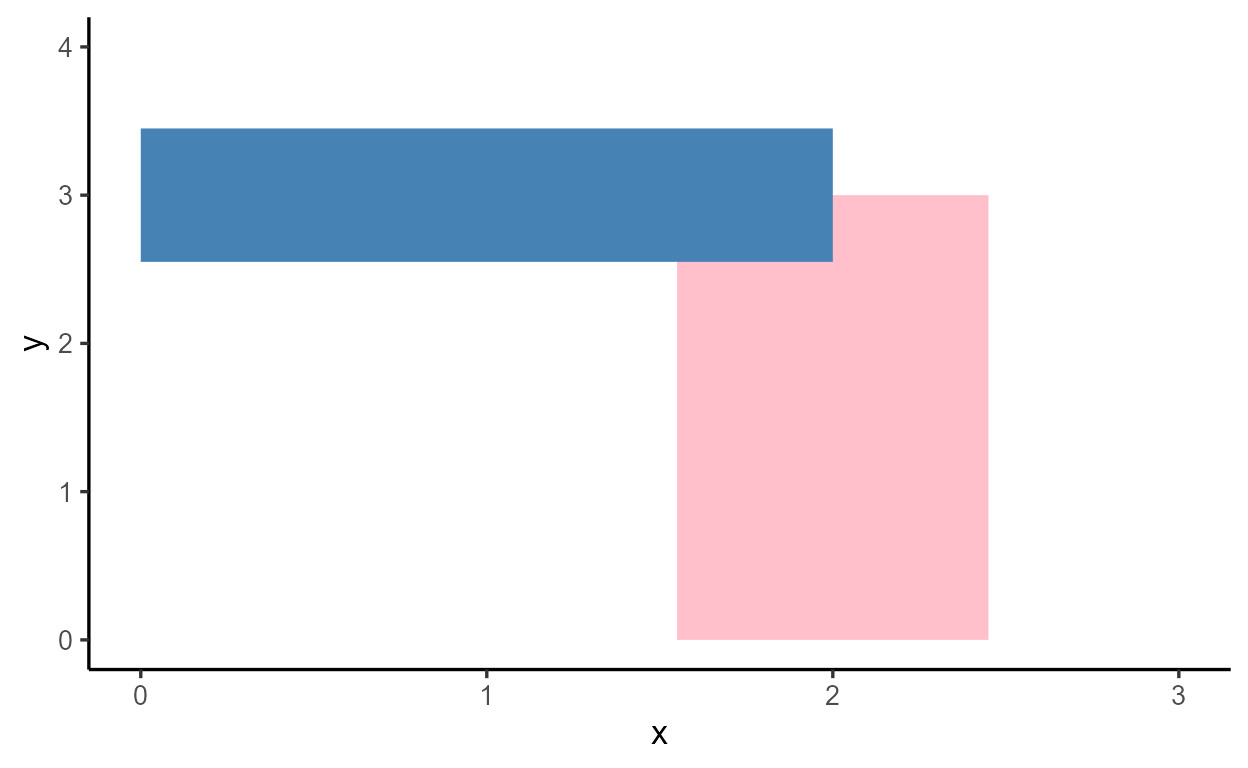## The `\$compute_*()` family of methods

Here, let’s look at how the statistical transformation is implemented in the `\$compute_*()` family of methods. The `p_bar2` ggplot is printed again below.

``````p_bar2
``````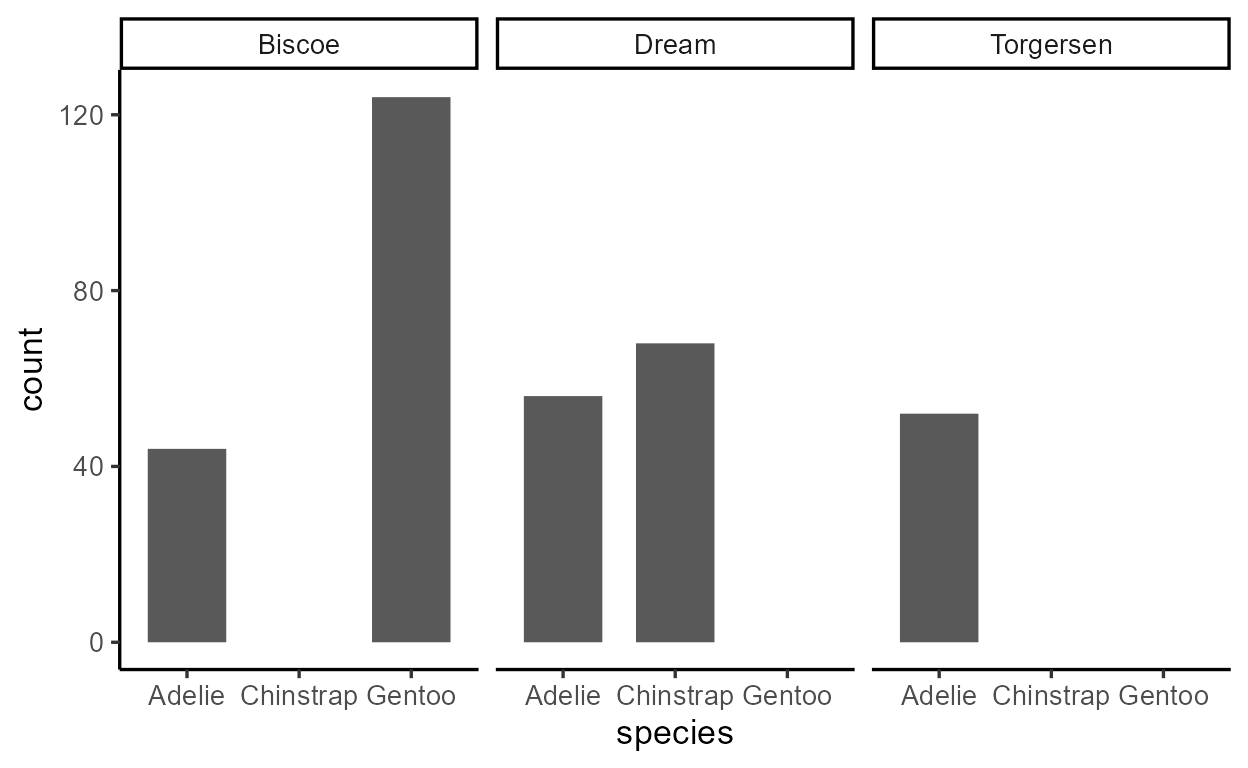The `geom_bar()` layer in our plot computes and draws five bars across three panels. This is reflected in the number of calls to the `\$compute_*()` methods:

• One call to `Stat\$compute_layer()` for our one bar layer
``````ggtrace_inspect_n(p_bar2, Stat\$compute_layer)
``````
``````   1
``````
• Three calls to `Stat\$compute_panel()` for three facets in the bar layer
``````ggtrace_inspect_n(p_bar2, Stat\$compute_panel)
``````
``````   3
``````
• Five calls to `StatCount\$compute_group()` for five bars across the three facets
``````ggtrace_inspect_n(p_bar2, StatCount\$compute_group)
``````
``````   5
``````

Keep in mind that there’s a hierarchy to how the `\$compute_*()` functions are called:

```  Stat\$compute_layer()
|---  Stat\$compute_panel()
|---  StatCount\$compute_group()
|---  StatCount\$compute_group()
|---  Stat\$compute_panel()
|---  StatCount\$compute_group()
|---  StatCount\$compute_group()
|---  Stat\$compute_panel()
|---  StatCount\$compute_group()
```

The `\$compute_*()` family of functions implement what’s called a split-apply-combine design. If you haven’t heard of it before, it’s basically like the “divide and conquer” strategy: you break down the problem to the smallest, most essential pieces, work on them individually, then bring them together into one solution.

Play around with the expandable nested tables below to get a sense of what kind of information is passed down to the `\$compute_group()` method, and what each `\$compute_group()` call returns for the bar layer in our plot:

### 1) Split

The `\$compute_layer()` method first splits up the data by facet and passes them down to `\$compute_panel()`. Then, the `\$compute_panel()` method splits up the data by group and passes them down to `\$compute_group()`.

### 2) Apply

The `\$compute_group()` method applies to each of the splits and returns a modified data:

### 3) Combine

The output of `\$compute_group()` calls are combined by panel and returned by `\$compute_panel()`, then combined again and returned by `\$compute_layer()`:

## The layer’s data frame representation

Remember how I said that the output of `Stat\$compute_layer()` for our `geom_bar()` layer is the dataframe representation of the layer at a particular stage in the pipeline? If this dataframe representation were to change, then it would affect how the layer gets drawn down the line.

We saw how `Stat*` extensions do this by changing how a ggproto method is defined (namely, via `\$compute_group()`). But we can also test this on-the-fly as well, without writing a whole ggproto extension ourselves.

For example, we can modify the output of `Stat\$compute_layer()` that we grabbed with `ggtrace_inspect_return()` before and save it to a new variable:

``````modified_compute_layer_output <- compute_layer_output %>%
mutate(count = count ^ 2) #< square the counts

modified_compute_layer_output
``````
``````    count prop x width flipped_aes PANEL group
1  1936    1 1   0.7       FALSE     1     1
2 15376    1 3   0.7       FALSE     1     3
3  3136    1 1   0.7       FALSE     2     1
4  4624    1 2   0.7       FALSE     2     2
5  2704    1 1   0.7       FALSE     3     1
``````

Then, we force `Stat\$compute_layer()` to return that modified dataframe instead when it’s called for `p_bar2`, by passing it to the `value` argument of `ggtrace_highjack_return()`:

``````ggtrace_highjack_return(
p_bar2, Stat\$compute_layer,
value = modified_compute_layer_output
)
``````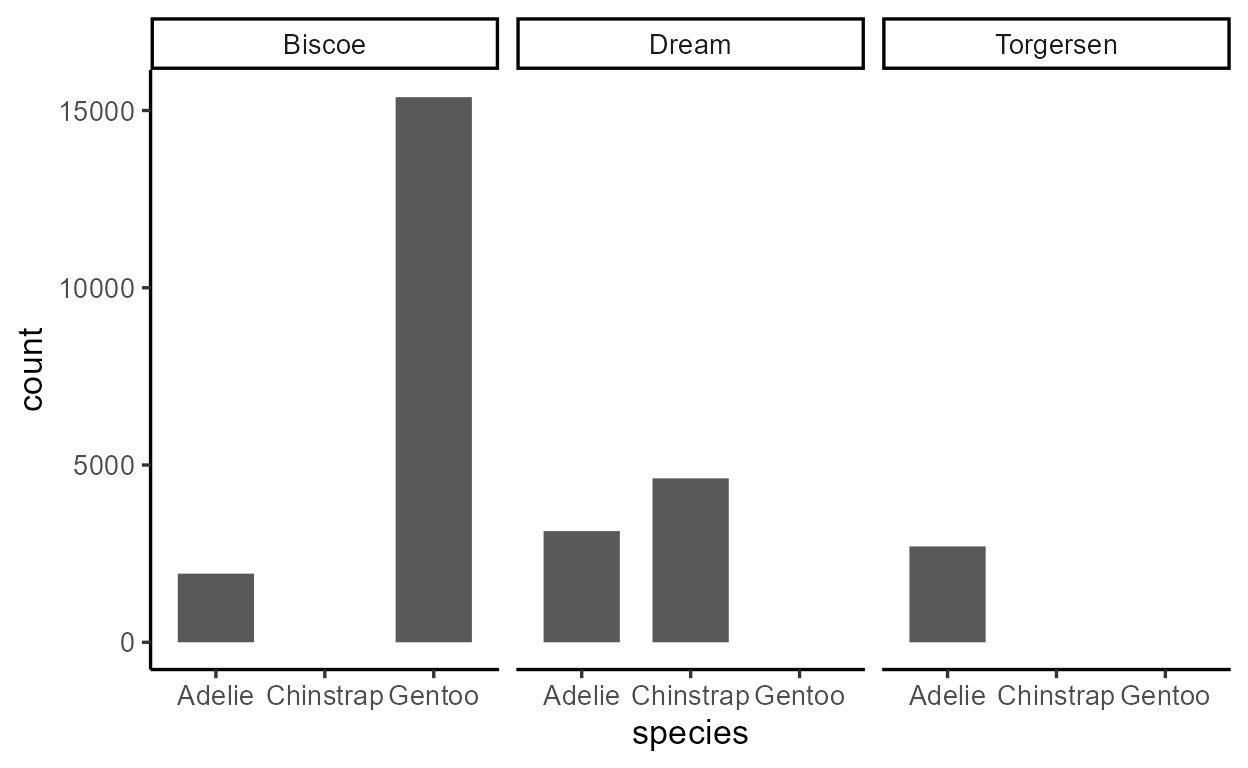See how this has direct consequences for our plot?

Here’s another one - we can modify the dataframe about to be returned by `StatCount\$compute_group()`, targeting just the third time it’s called, with `cond = 3`. This time we do the modification in place by passing an expression to the `value` argument, where `returnValue()` evaluates to the value about to be returned by the method:

``````ggtrace_highjack_return(
p_bar2, StatCount\$compute_group, cond = 3,
value = quote({
returnValue() %>%
mutate(count = count ^ 2)
})
)
``````Again, a big consequence for the plot down the line.

The lesson here is that the dataframe representation of the layer undergoes incremental updates in the internals, gradually working up to its final drawing-ready form that we can see using `layer_data()`.

``````layer_data(p_bar2)
``````
``````      y count prop x flipped_aes PANEL group ymin ymax xmin xmax colour   fill
1  44    44    1 1       FALSE     1     1    0   44 0.65 1.35     NA grey35
2 124   124    1 3       FALSE     1     3    0  124 2.65 3.35     NA grey35
3  56    56    1 1       FALSE     2     1    0   56 0.65 1.35     NA grey35
4  68    68    1 2       FALSE     2     2    0   68 1.65 2.35     NA grey35
5  52    52    1 1       FALSE     3     1    0   52 0.65 1.35     NA grey35
size linetype alpha
1  0.5        1    NA
2  0.5        1    NA
3  0.5        1    NA
4  0.5        1    NA
5  0.5        1    NA
``````

In this sense, ggproto methods are like functions that intervene at different steps of what is essentially a data wrangling pipeline, making piecemeal changes to the layer data. Consequently, the work of a single ggproto method can have far reaching consequences for the plot down the line.

I promise this is the end of the `{ggtrace}` self-promo, but I encourage you to play around with the internals using the workflow functions from the package - it can help refine your intuitions about how ggplot internals work, with low barrier and no risk. Check out the package website if you’d like to know more.

## Other `\$compute_*()` extensions

Before we wrap up, I have to come clean - I’ve actually been misleading you in instilling this divide between `\$compute_layer()` and `\$compute_panel()` on one hand, and `\$compute_group()` on the other. Of course, `\$compute_group()` is the most natural extension point, but nothing stops you from doing the necessary calculations in `\$compute_layer()` or `\$compute_panel()` instead.

In fact, there are several circumstances where you don’t want to do things at the group level:

The first reason is for efficiency. Consider the case of `geom_point()` - it’s a layer that draws points from `x` and `y` values, as is. The stat ggproto for this layer is `StatIdentity`, and all it does in the `\$compute_*()` step is to return the data as it received it.

``````class( geom_point()\$stat )
``````
``````   "StatIdentity" "Stat"         "ggproto"      "gg"
``````

One way of implementing this is to define a `StatIdentity\$compute_group()` that just returns `data`, but this is unnecessarily complex - you’re still splitting the data by panel and group, only to not do anything with it.

Therefore, the appropriate extension point is actually `\$compute_layer()` - you return the data as soon as you receive it, without forwarding the data to `\$compute_panel()` and `\$compute_group()`. Indeed, that’s how `StatIdentity` is actually implemented.

``````get_method_inheritance(StatIdentity)
``````
``````  \$Stat
 "aesthetics"      "compute_group"   "compute_panel"   "default_aes"
 "extra_params"    "finish_layer"    "non_missing_aes" "optional_aes"
 "parameters"      "required_aes"    "retransform"     "setup_data"
 "setup_params"

\$StatIdentity
 "compute_layer"
``````
``````StatIdentity\$compute_layer
``````
``````  function (self, data, params, layout)
{
data
}
<bytecode: 0x000002e4a4084350>
<environment: namespace:ggplot2>
``````

A second reason is that sometimes you need to do calculations at the panel level, not the group level. This is the case for `StatUnique`. It’s an uncommon stat that’s mainly used to deal with overplotted text by removing duplicates in the dataframe representation of the layer.5

It’s subtle (you need to zoom in to the plots to see), but there’s a difference in quality between overlapping text on the left (with the `StatIdentity` default) versus the solution on the right with `StatUnique`.

``````tibble(x = rep(1, 50), y = x) %>%
ggplot(aes(x, y)) +
geom_text(
aes(label = "Some Text"),
size = 25, fontface = "bold",
stat = StatIdentity #< default
)
``````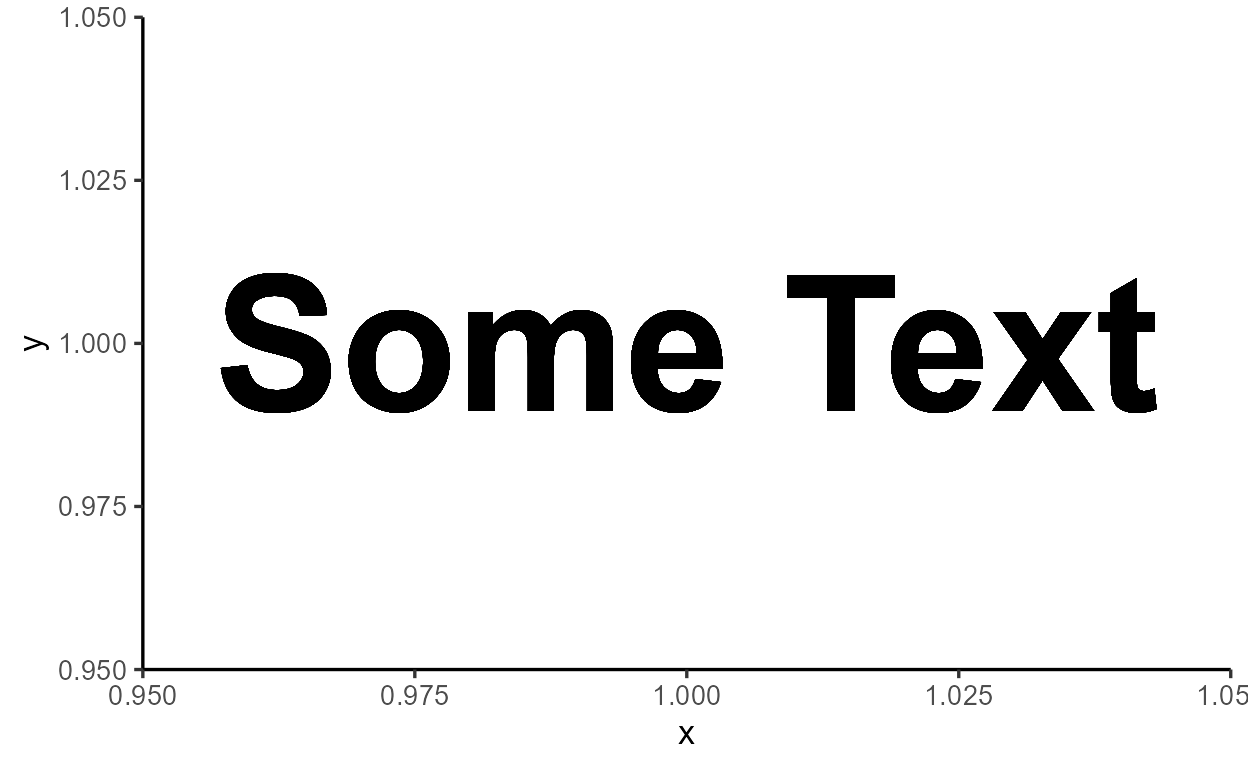``````tibble(x = rep(1, 50), y = x) %>%
ggplot(aes(x, y)) +
geom_text(
aes(label = "Some Text"),
size = 25, fontface = "bold",
stat = StatUnique
)
``````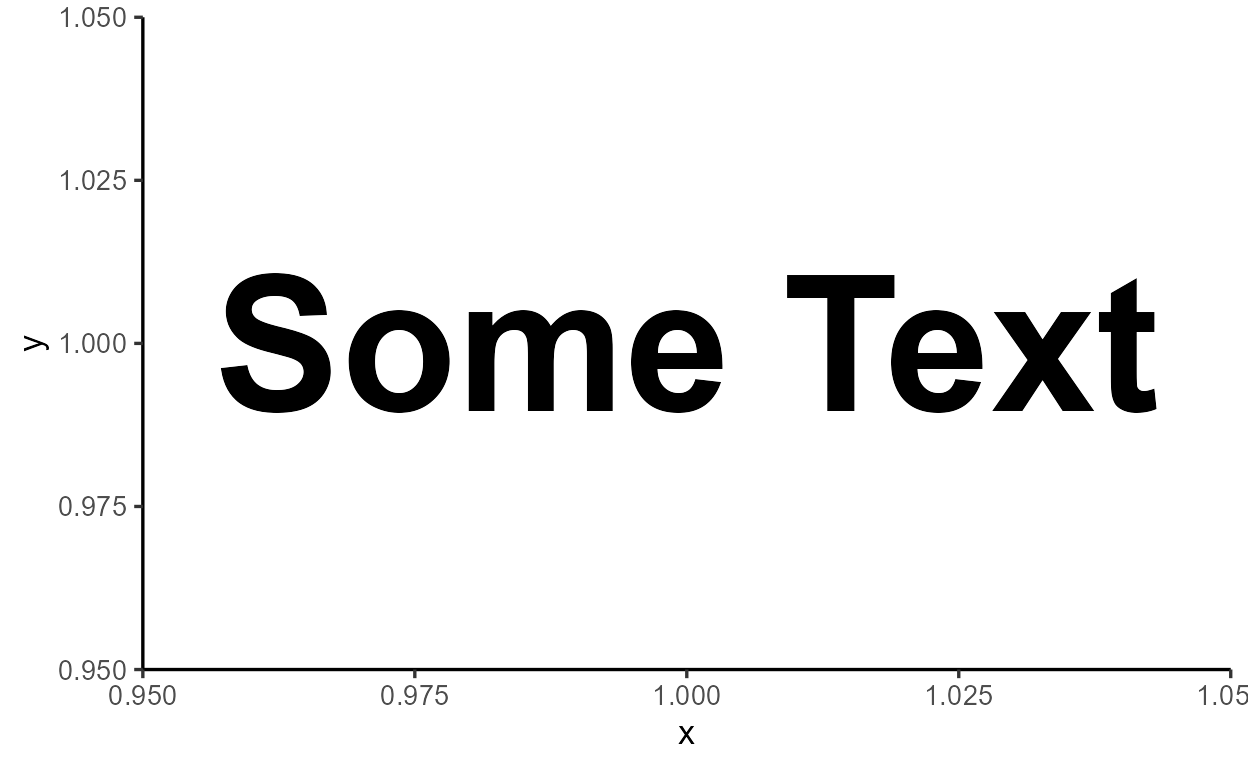Figure 1: Zoomed in to the letter “S” from the two plots.

You might think that `StatUnique\$compute_layer()` is returning `unique(data)` at the layer level, but consider this behavior of `StatUnique`:

``````tibble(x = rep(1, 50), y = rep(1, 50),
g = rep_len(LETTERS[1:4], 50)) %>%
ggplot(aes(x, y)) +
geom_text(
aes(label = "Some Text"),
size = 10, fontface = "bold",
stat = StatUnique
) +
facet_wrap(~ g)
``````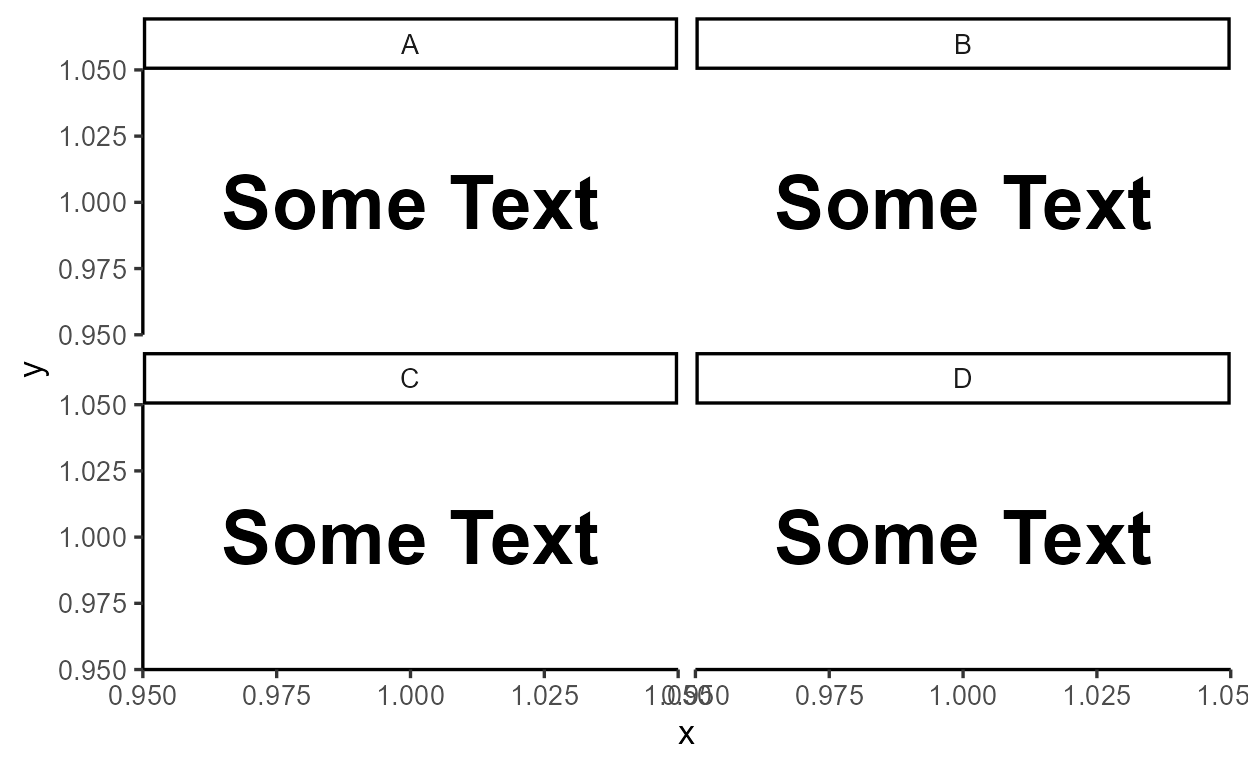We see that `StatUnique` doesn’t remove just any data point duplicates - it removes duplicates within each facet. Thus, `unique(data)` is implemented inside `StatUnique\$compute_panel()`, which is more in line with the goal of preventing visually overlapping text.

``````get_method_inheritance(StatUnique)
``````
``````  \$Stat
 "aesthetics"      "compute_group"   "compute_layer"   "default_aes"
 "extra_params"    "finish_layer"    "non_missing_aes" "optional_aes"
 "parameters"      "required_aes"    "retransform"     "setup_data"
 "setup_params"

\$StatUnique
 "compute_panel"
``````
``````StatUnique\$compute_panel
``````
``````  function (data, scales)
unique(data)
<bytecode: 0x000002e4a28c4838>
<environment: namespace:ggplot2>
``````

Lastly, there are rare cases of where you’d want to extend multiple `\$compute_*()` methods at once. For example, `StatYdensity` is used by `geom_violin()` to calculate the size and shape of violins, and it extends both `\$compute_panel()` and `\$compute_group()`:

``````class( geom_violin()\$stat )
``````
``````   "StatYdensity" "Stat"         "ggproto"      "gg"
``````
``````get_method_inheritance(StatYdensity)
``````
``````  \$Stat
 "aesthetics"    "compute_layer" "default_aes"   "finish_layer"
 "optional_aes"  "parameters"    "retransform"   "setup_data"

\$StatYdensity
 "compute_group"   "compute_panel"   "extra_params"    "non_missing_aes"
 "required_aes"    "setup_params"
``````

This is done to create an effect where densities are calculated per group, and then scaled within each facet. Note how violin areas are equal within a facet but not across facets.6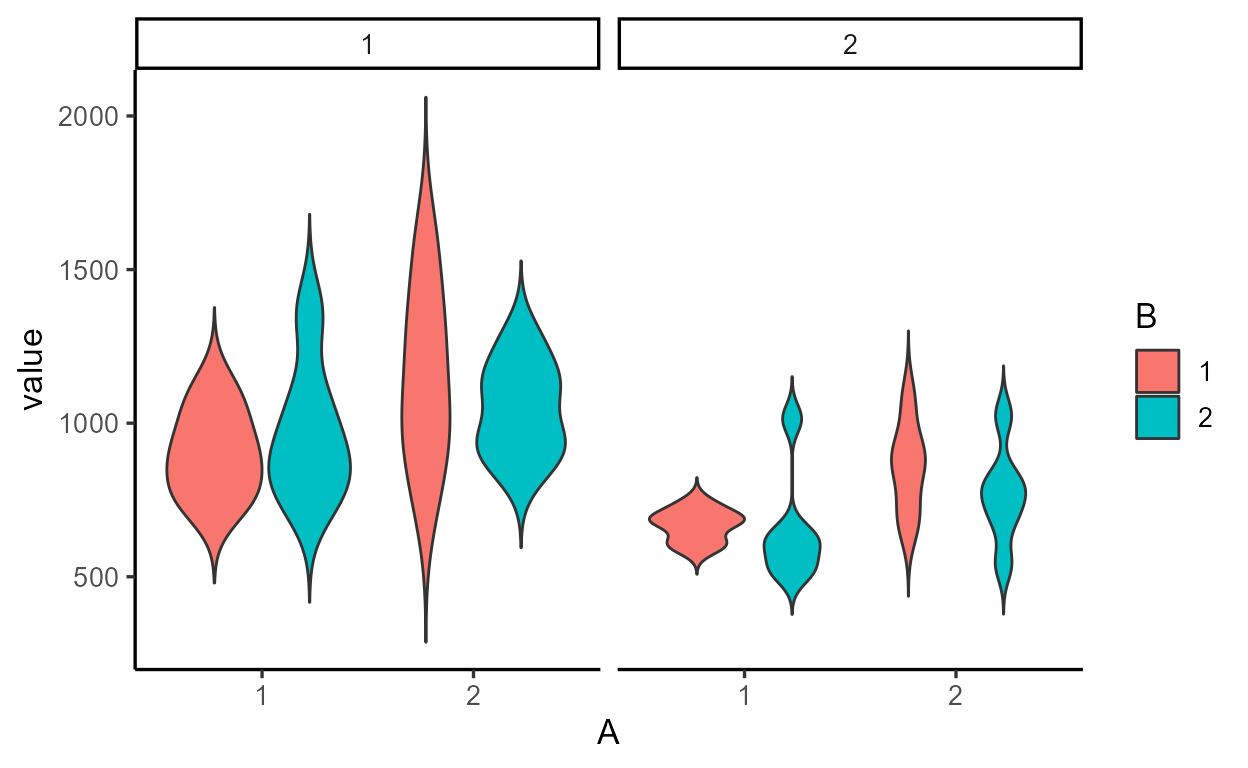## Conclusion

In Part 1, we were introduced to this idea of there being a data frame representation for each layer, which gets updated and augmented over the course of rendering a ggplot. We saw how one of the changes to the layer data is the statistical transformation whereby new variables like `count` are computed internally and become available for delayed aesthetic mapping using `after_stat()`. We saw how this statistical transformation step isn’t as scary as it looks - it looked like a standard data wrangling procedure that we could express with `group_by()` and `summarize()`.

The goal of Part 2 was to expose the exact details of the statistical transformation step. We had our first encounter with ggproto methods which we can think of as data wrangling functions that live inside lists. We saw how a family of `\$compute_*()` methods called by-layer, by-facet, and by-group implement a split-apply-combine process much like the `group_by()` + `summarize()` combo. We also learned that the motivation behind this odd-looking ggproto system is to support an extension mechanism that allows us to subclass new `Stat*` and `Geom*` ggprotos that define their own custom behavior for a method like `\$compute_group()`.

Sorry if Part 2 was a bit too theoretical! In Part 3, we’ll leave this whole ggproto thing behind to talk about `after_scale()` and `stage()`, two more delayed aes eval functions.

## Sneak peak of Part 3

I’ll leave you with two examples as a teaser:

Example 1: `after_scale()` is like `after_stat()`, but targets the data after the (non-positional) scale transformation step, which happens towards the end of the build pipeline:

``````library(colorspace)
p_boxplot <- penguins %>%
filter(!is.na(flipper_length_mm)) %>%
ggplot(aes(x = species, y = flipper_length_mm, fill = species)) +
geom_boxplot(
aes(color = after_scale(darken(fill, .5))),
width = .4
) +
theme_classic()
p_boxplot
``````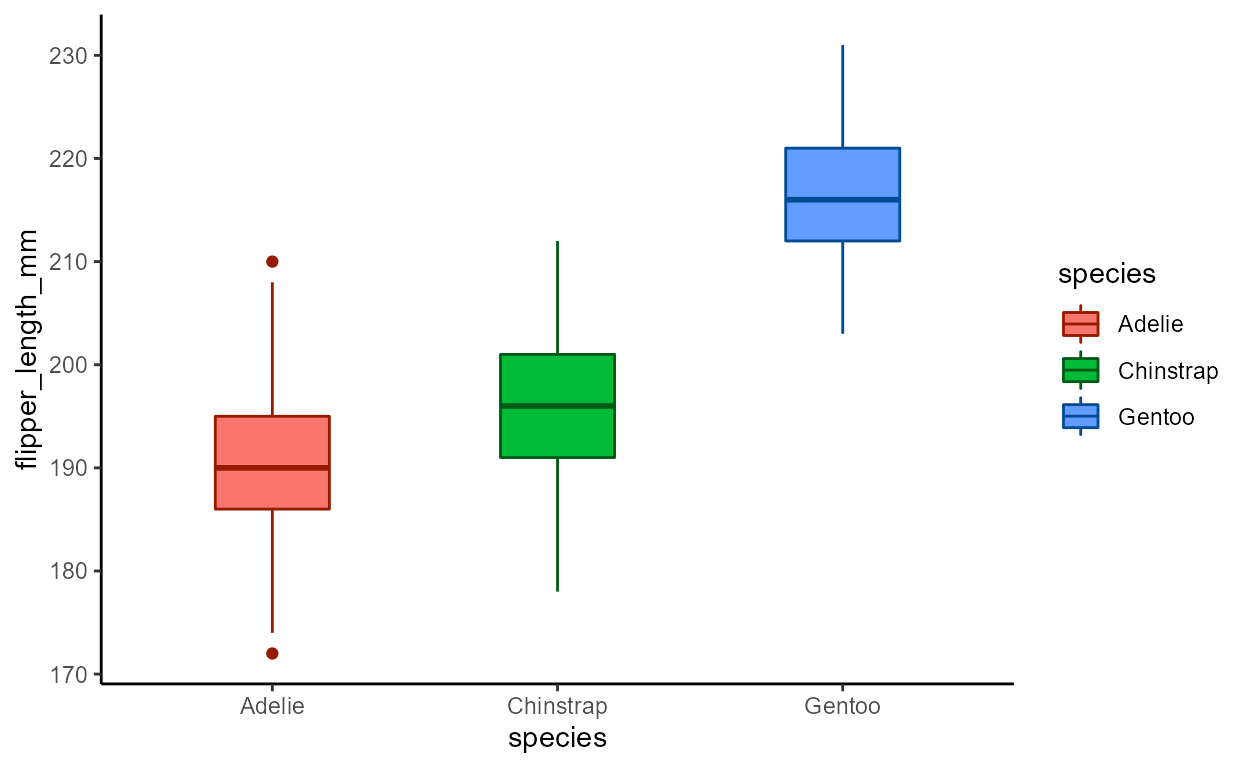Example 2: `stage()` allows you to re-map to the same aesthetic using variables from different points in the build pipeline.

``````# Generates warning in v3.3.6; fixed in dev version (PR #4707)
p_boxplot +
geom_text(
aes(y = stage(flipper_length_mm, c(lower, middle, upper)),
label = after_stat(c(lower, middle, upper)),
color = after_scale(darken(fill, .8))),
size = 4, hjust = 1,
position = position_nudge(x = .5),
stat = StatBoxplot
) +
theme_classic()
``````## A taste of writing ggproto extensions

Though writing ggproto extensions is beyond the scope of this blog series,7 we’re now well prepared for it after working through the logic of how the top-level template `Stat` ggproto gets extended in child `Stat*` ggprotos like `StatCount`. All that’s missing is the syntax that implements this (that’s usually the easy part!).

Just to give you a taste without going into it too deep, this is an example stat extension inspired by Gina Reynolds that calculates an internal variable called `rowid` inside `\$compute_layer()` and also sets a default aesthetic mapping of `label = after_stat(rowid)` in `\$default_aes`:

``````StatRowID <- ggplot2::ggproto(

# Create a new ggproto of class "StatRowID"
`_class` = "StatRowID",
# That inherits from the top-level `Stat` ggproto
`_inherit` = Stat,

# Extension point: add a `rowid` column to the data at layer-level
compute_layer = function(self, data, params, layout) {
data\$rowid <- seq_len(nrow(data))
data
},

# Extension point: map the computed `rowid` variable to `label`
default_aes = aes(label = after_stat(rowid))

)

tibble(x = runif(20), y = runif(20), g = rep(c("A", "B"), each = 10)) %>%
ggplot(aes(x, y, fill = g)) +
geom_label(stat = StatRowID) # or "RowID"
``````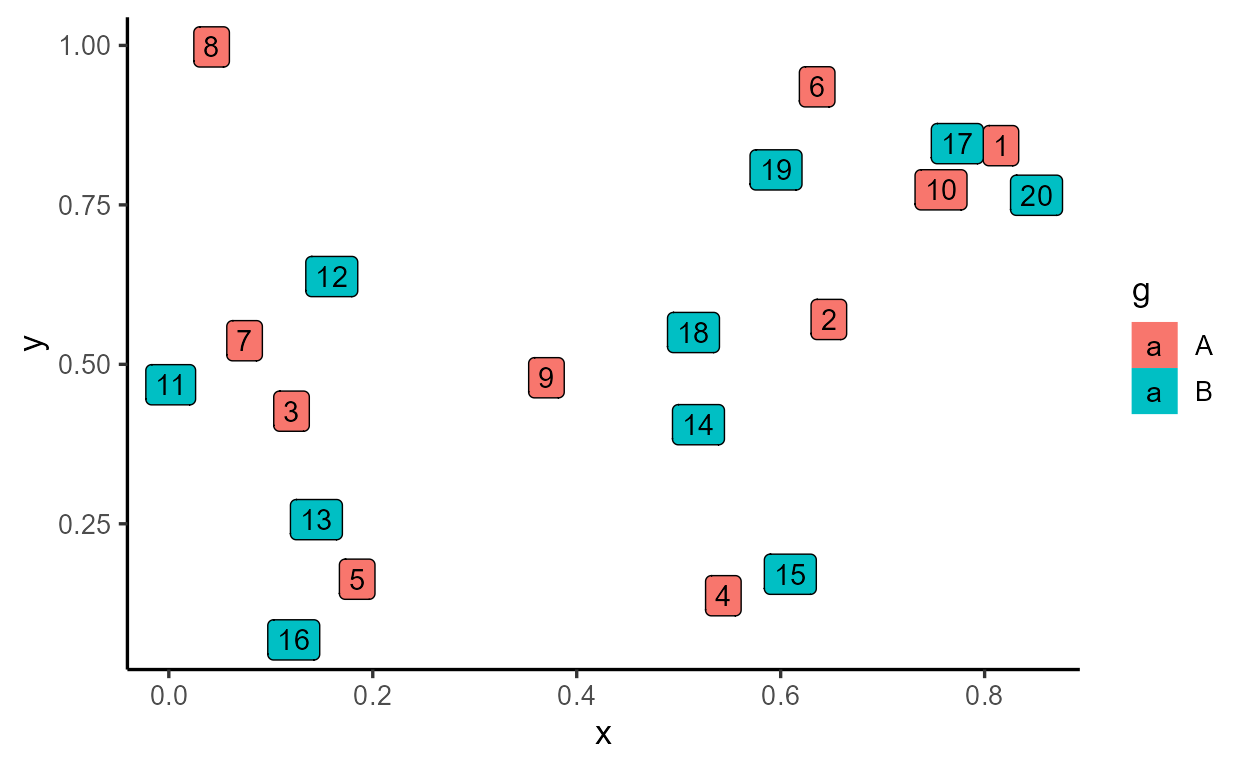If we wanted row IDs to be calculated by group, we simply move the computation to `\$compute_group()`

``````StatRowIDbyGroup <- ggplot2::ggproto(
`_class` = "StatRowIDbyGroup",
`_inherit` = Stat,
default_aes = aes(label = after_stat(rowid)),
# Extend `compute_group` instead of `compute_layer`
compute_group = function(self, data, scales) {
data\$rowid <- seq_len(nrow(data))
data
}
)

tibble(x = runif(20), y = runif(20), g = rep(c("A", "B"), each = 10)) %>%
ggplot(aes(x, y, fill = g)) +
geom_label(stat = StatRowIDbyGroup) # or "RowIDbyGroup"
``````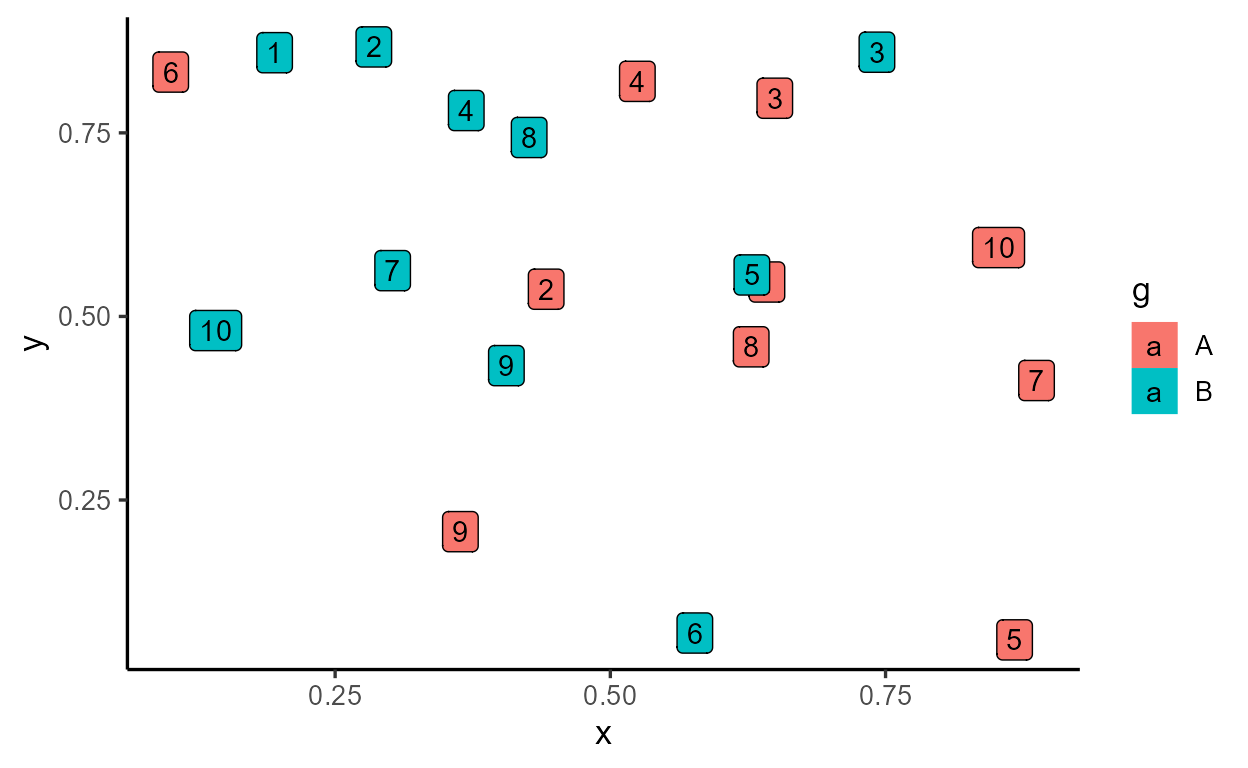If the sensible use of `statRowIDbyGroup` is for it to be paired with the label geom, then we write a layer function (also called a constructor function) that wraps around `ggplot2::layer()`. Inside `stat_rowid()`, we hard code `stat = StatRowIDbyGroup` and expose the `geom = "label"` default argument to the user:

``````stat_rowid <- function(mapping = NULL, data = NULL,
geom = "label", position = "identity",
..., na.rm = FALSE, show.legend = NA, inherit.aes = TRUE) {
ggplot2::layer(
# All layers need data and aesthetic mappings
data = data, mapping = mapping,
# The layer's choice of Stat, Geom, and Position
stat = StatRowIDbyGroup, geom = geom, position = position,
# Standard parameters available for all `layer()`s (there are more)
show.legend = show.legend, inherit.aes = inherit.aes,
# Arguments to be passed down to Stat/Geom/Position
params = list(na.rm = na.rm, ...)
)
}

tibble(x = runif(20), y = runif(20), g = rep(c("A", "B"), each = 10)) %>%
ggplot(aes(x, y, fill = g)) +
stat_rowid()
``````By following these design principles, you get a lot of features for free, like the ability to swap out the geom and map the internally calculated `rowid` variable elsewhere via `after_stat()`:

``````tibble(x = runif(20), y = runif(20), g = rep(c("A", "B"), each = 10)) %>%
ggplot(aes(x, y, color = g)) +
stat_rowid(
aes(
size = after_stat(rowid),
alpha = after_stat(rowid/max(rowid))
),
geom = "text"
)
``````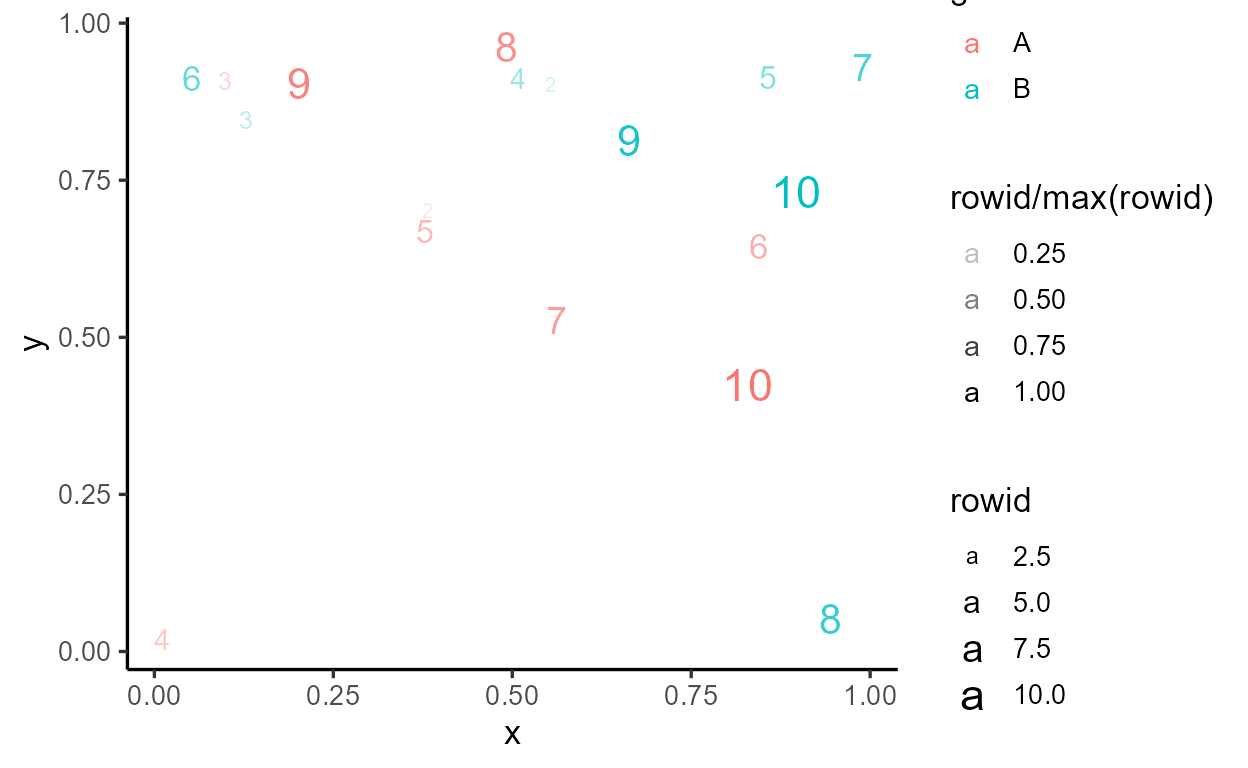If you’re feeling ambitious, I highly recommend starting with Thomas Lin Pedersen’s rstudio::conf talk. Good luck!

1. Technically, it was returning the output of `ggplot2:::Layer\$compute_statistic()` which returns the `\$compute_layer()` method called by the layer’s stat. But we don’t need to worry about this distinction for this blog post.↩︎

2. Missing columns get added back in when `\$compute_panel()` combines the output of `\$compute_group()` calls.↩︎

3. For example, one big difference is that parameters are passed in as a list to the `params` argument of `compute_layer()`, which is then spliced and passed in as the `...` of `compute_panel()` through `do.call()`.↩︎

4. It’s actually optional and only available if the method specifies an argument called `self`. As long as it’s present in the formals, it can appear in any position. The convention is to define all ggproto methods with `self` as the first argument.↩︎

5. An alternative solution that I personally prefer is `annotate(geom = "text", ..)`.↩︎

6. I’m not sure about the rationale for this, and people have brought up wanting to change this default. Given everything we’ve discussed so far, we don’t have to read the source code to know that if you want all violins in the layer to have the same size, we should move the calculations in `\$compute_panel()` to `\$compute_layer()` instead.↩︎

7. Other resources exist for that (in fact, most resources on learning the internals focus on writing extensions). Chapter 20 and 21 of the ggplot2 book is a good place to start.↩︎